# ACT Math : How to find the solution of a rational equation with a binomial denominator

## Example Questions

### Example Question #1 : Binomial Denominators

For the equation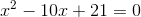, what is(are) the solution(s) for?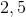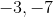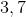Explanation:, can be factored to (x -7)(x-3) = 0. Therefore, x-7 = 0 and x-3 = 0. Solving for x in both cases, gives 7 and 3.

### Example Question #2 : Binomial Denominators

Simplify: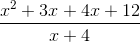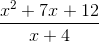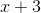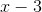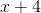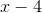Explanation:

In order to begin this kind of a problem, it's key to look at parts of the rational expression that can be simplified.

In this case, the denominator is an already-simplified binomial; however, the numerator can be factored through "factoring by grouping." This can be a helpful idea to keep in mind when you come across a polynomial with four terms and simplifying is involved.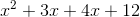can be simplified first by removing the common factor offrom the first two terms and the common factor offrom the last two terms: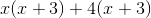This leaves two terms that are identical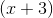and their coefficients, which can be combined into another term to complete the factoring: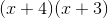Consider the denominator; the quantity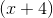appears, so thein the numerator and in the denominator can be cancelled out. The simplified expression is then left as.

### Example Question #1 : How To Find The Solution Of A Rational Equation With A Binomial Denominator

Simplify: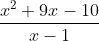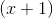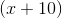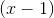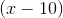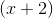Explanation:

In order to begin this kind of a problem, it's key to look at parts of the rational expression that can be simplified.

In this case, the denominator is an already-simplified binomial; however, the numerator can be factored.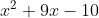The roots will be numbers that sum up tobut have the product of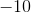.

The options include: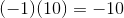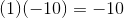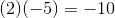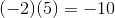When these options are summed up: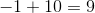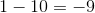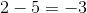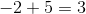We can negate the last three options because the first option ofandfulfill the requirements. Therefore, the numerator can be factored into the following: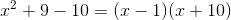Because the quantityappears in the denominator, this can be "canceled out." This leaves the final answer to be the quantity.